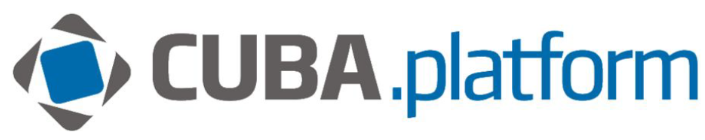# Graph percentage axis

Hi,

How can I add some percentages on the chart ?
Let’s say I have a datasource :

``````select e.month, sum(e.profit), sum(e.sales), sum(e.profit)/sum(e.sales) from carrp\$profitData e
group by e.month``````

and the properties :

``````
<properties>
<property datatype="string"
name="month"/>
<property datatype="int"
name="profit"/>
<property datatype="int"
name="sales"/>
<property datatype="double"
name="percentRatio"/>
</properties>
``````

Actually I want to have the percentage of sum(e.profit)/sum(e.sales)
In axes I have the unit="%" but it show 0 in the graph.

It’s this coming from the

``sum(e.profit)/sum(e.sales)``

, if yes is there any solution to do this directly in graph?

Hi,

When dividing one integer into another, an integer division is obtained and the fractional part is discarded. Cast one of the values to a fractional number and that will fix your issue, e.g.

``````select e.month, sum(e.profit), sum(e.sales),  CAST(sum(e.profit) NUMERIC(10,2))/sum(e.sales) from carrp\$profitData e
group by e.month``````

Regards,
Gleb

Hi Gleb,

Thanks a lot! that was very helpful!

regards,
Gent